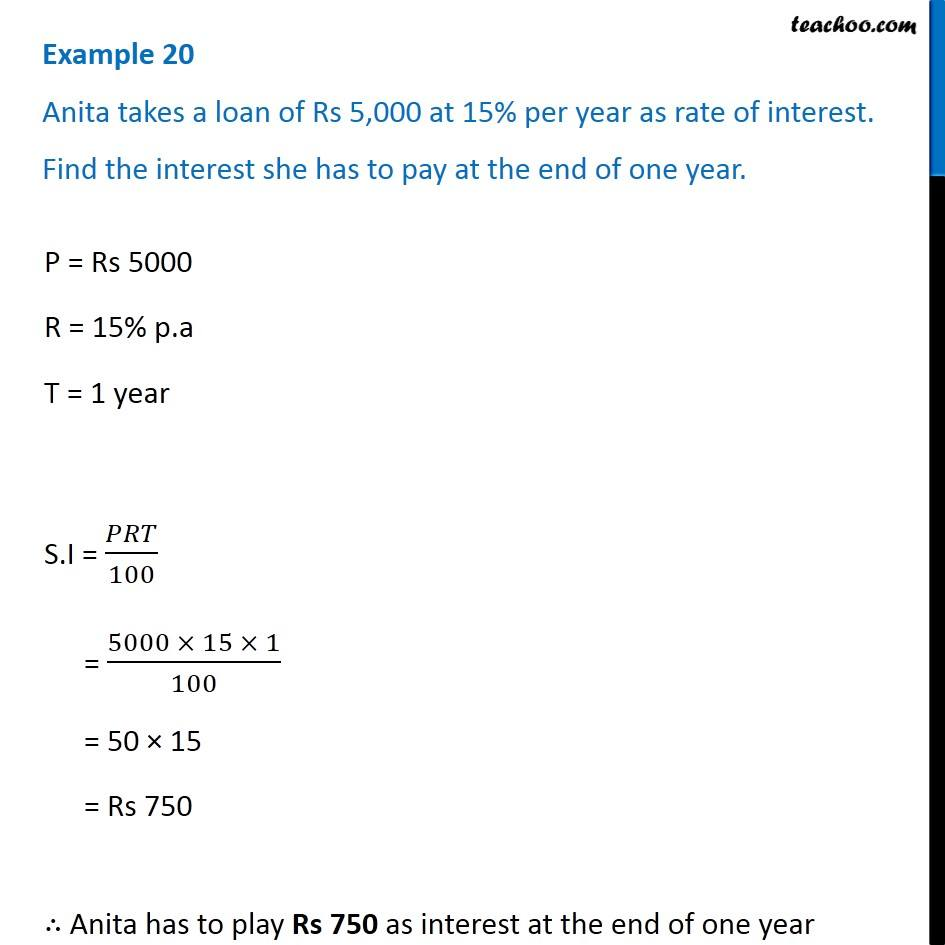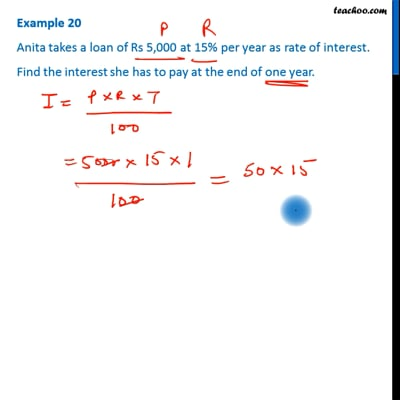Examples

Chapter 8 Class 7 Comparing Quantities
Serial order wiseThis video is only available for Teachoo black users

Get live Maths 1-on-1 Classs - Class 6 to 12

### Transcript

Example 20 Anita takes a loan of Rs 5,000 at 15% per year as rate of interest. Find the interest she has to pay at the end of one year. P = Rs 5000 R = 15% p.a T = 1 year S.I = 𝑃𝑅𝑇/100 = (5000 × 15 × 1)/100 = 50 × 15 = Rs 750 ∴ Anita has to play Rs 750 as interest at the end of one year